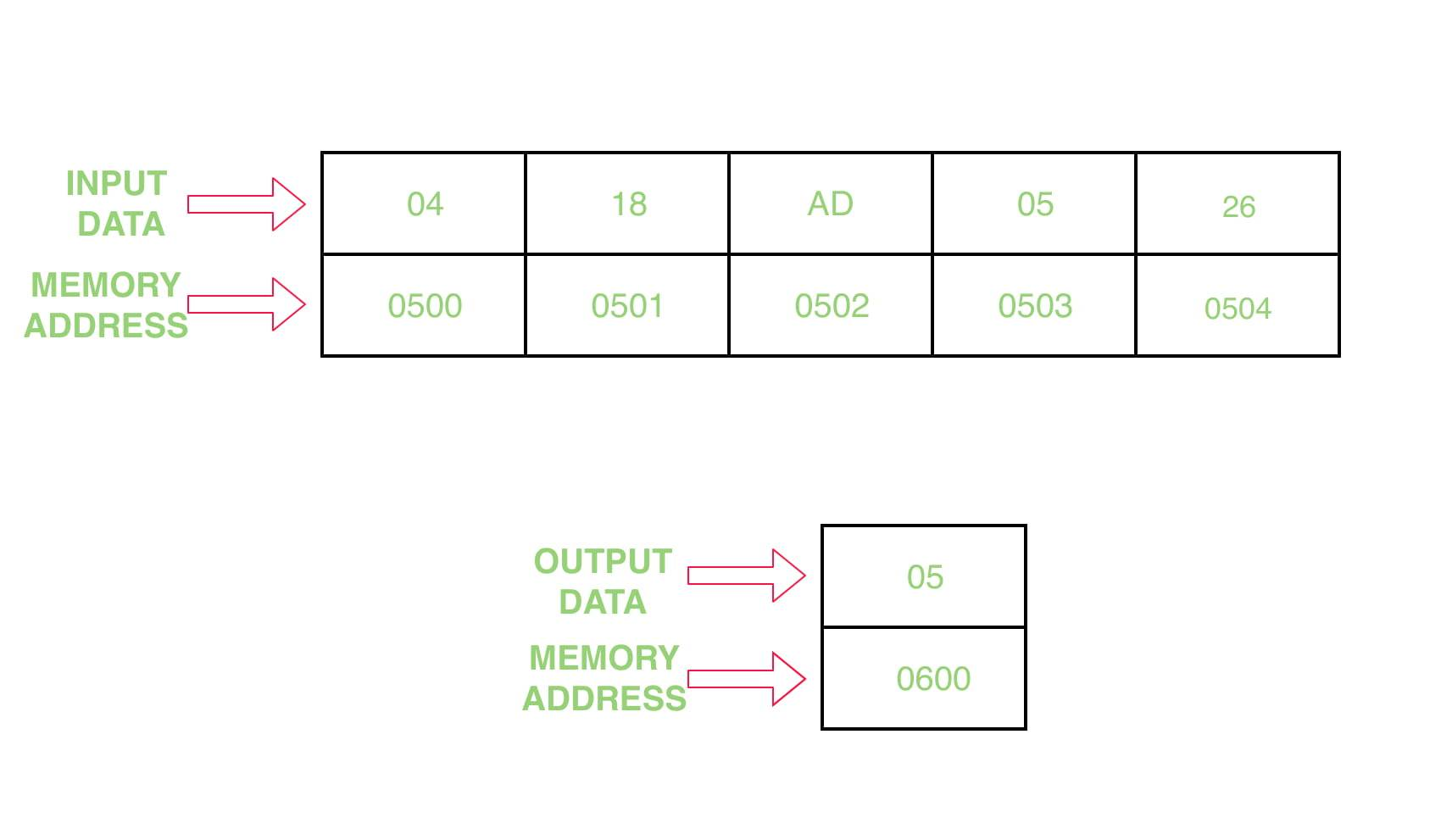# 8086 program to find the min value in a given array

Problem – Write a program to find the min value in a given array in assembly 8086 microprocessor

Example –Assumptions – Starting address of input array is 0500 and and store the result at address 0600

Algorithm –

1. Assign value 500 in SI and 600 in DI
2. Move the contents of [SI] in CL and increment SI by 1
3. Assign the value 00 H to CH
4. Move the content of [SI] in AL
5. Decrease the value of CX by 1
6. Increase the value of SI by 1
7. Move the contents of [SI] in BL
8. Compare the value of BL with AL
10. Move the contents of BL in AL
11. Jump to step 6 until the value of CX becomes 0, and decrease CX by 1
12. Move the contents of AL in [DI]
13. Halt the program

Program –

0400 MOV SI, 500 SI <- 500
0403 MOV DI, 600 DI <- 600
0406 MOV CL, [SI] CL <- [SI]
0408 MOV CH, 00 CH <- 00
040A INC SI SI <- SI+1
040B MOV AL, [SI] AL <- [SI]
040D DEC CX CX <- CX-1
040E INC SI SI <- SI+1
040F MOV BL, [SI] BL <- [SI]
0411 CMP AL, BL AL-BL
0413 JC 0417 Jump if carry is 1
0415 MOV AL, BL AL <- BL
0417 LOOP 040E Jump if CX not equal to 0
0419 MOV [DI], AL [DI] <- AL
041B HLT End of the program

Explanation –

1. MOV SI, 500 assigns 500 to SI
2. MOV DI, 600 assigns 600 to DI
3. MOV CL, [SI] moves the content of [SI] to CL register
4. MOV CH, 00 assign 00 to CH register
5. INC SI increase the value SI by 1
6. MOV AL, [SI] moves the content of [SI] to AL register
7. DEC CX decrease the content of CX register by 1
8. INC SI increase the value SI by 1
9. MOV BL, [SI] moves the content of [SI] to BL register
10. CMP AL, BL subtract the value of BL register from AL and it modify flag registers
12. MOV AL, BL moves the content of BL register to AL register
13. LOOP 040E runs loop till CX not equal to Zero and decrease the value of CX by 1
14. MOV [DI], AL moves the content of AL to [DI]
15. HLT stops the execution of program

Attention reader! Don’t stop learning now. Get hold of all the important CS Theory concepts for SDE interviews with the CS Theory Course at a student-friendly price and become industry ready.

My Personal Notes arrow_drop_upCheck out this Author's contributed articles.

If you like GeeksforGeeks and would like to contribute, you can also write an article using contribute.geeksforgeeks.org or mail your article to contribute@geeksforgeeks.org. See your article appearing on the GeeksforGeeks main page and help other Geeks.

Please Improve this article if you find anything incorrect by clicking on the "Improve Article" button below.